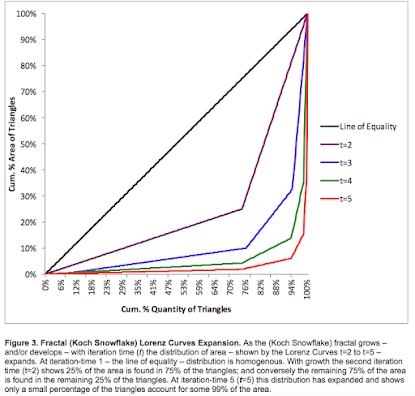## Posts

Showing posts from January, 2011

### Fractal equilibrium countContinuing on from my earlier blog on fractal equilibrium : Koch Curve Animation From a fixed view point: all fractals ('attractors')  form their shape (are at fractal equilibrium) at and around 7 plus or minus 2  iterations - any more than this will come at too high a cost, and with no extra benefit - as shown in the animation of the Koch Snowflake development above. The 5 iterations to develop the fractal Koch snowflake in fig. 1 (below) - the point where the blue extra (Marginal) area (MA) and green extra (Marginal) cost (MC) intersect, corresponds with where the shape of the snowflake is fully developed.  This,  I believe, is not only a demonstration but is an explanation for  The Magical Number - Seven, Plus or Minus Two , but is also observable through-out our reality. From any stand-point, there will be around 4,5,6,7,or 8 levels of protrusion. For example, from where I am writing, I can see out my window where there is a park and some buildings. The building

### Fractal: Equilibrium Perfect Knowledge and OutputFractal Equilibrium: This entry follows on from 'production of the fractal'. Here I am suggesting that equilibrium - in any sense - is a fundamental of the fractal. Koch Snowflake development: source, Wikipedia The above animation shows the development of the fractal, at iteration 5 or 6 fractal equilibrium is reached - where the shape (of the snowflake) is made; or where benefit production is equal to cost of production. Fig. 2b below, shows a closeup analysis of the fractal equilibrium, at least from a static point of view.  MC  intersects, or is equal to MA, at iteration 5 where the Area is equal to 1, due to the reciprocal of 1 itself being equal 1. Equilibrium - Perfect Knowledge* http://en.wikipedia.org/wiki/Perfect_information Any iteration less than fractal equilibrium will result in an imperfect (snowflake) shape or incomplete knowledge or information. Any iteration point greater fractal equilibrium will result with little added gain in informa

### Marginal Cost - Production of the FractalMarginal Cost - derived from the fractal Original Post The fractal demonstrates cost. To demonstrate the increasing cost - in effort and time - at each iteration (see my first blog on marginal analysis and the fractal)  to produce the fractal, I decided to use the the reciprocal and invert the Marginal Area, MA. The rationale for this choice is based on: the more area to the snowflake, the more the cost. I am sure there are other ways of doing this, but I figured that this method is as simple and as easy as calculating the MC again, it should be okay. Fig. 2 shows the original MA TA diagram with the MC. Background One thing about computer generated fractals is that they appear to be produced with ease, we simply forget that they would not be viewable if it were not for the memory and processing power of our modern computer. To see how complex and slow they are to draw one only has to take a pen and paper and have a go at drawing the Koch snowflake progression - step by s

### Marginal Analysis of the FractalMarginalism and Marginal Analysis : derived from the fractal. Here I preform basic marginal analysis on the Koch Snowflake Update May 2017 This is by far my best idea; I have written it up in a working paper at  my academia.edu  and  vixra , and named it:   Quantum Mechanics, Information and Knowledge, all Aspects of Fractal Geometry and Revealed in an Understanding of Marginal Economics. I shall post the Abstract, followed by the original post. I hope to have some collaborate and review my work in time. Abstract Fractal geometry is found universally and is said to be one of the best descriptions of our reality – from clouds and trees, to market price behaviour. As a fractal structure emerges  – the repeating of a simple rule –  it appears to share direct properties familiar to classical economics, including production, consumption, and equilibrium. This paper was an investigation into whether the mathematical principles behind ‘the market’ – known as marginalism – is an a

### Lorenz Curve of the Koch Snowflake FractalUpdate 2015, I have published/posted. I have found that Lorenz distribution is a fractal phenomenon. That the fractal models the Lorenz Curve and Gini coefficients increase as the fractal grows and develops. The distribution between groups accelerates with growth and development. Lorenz distribution is universal: income and wealth inequality is one aspect of a universal phenomenon and is scale-invariant.   https://www.academia.edu Demonstrating_Lorenz_Wealth_Distribution_and_Increasing_Gini_Coefficient_with_the_Iterating_Koch_Snowflake_Fractal_Attractor Also see:  Improved Fractal Lorenz Curve Wealth Distribution: a (universal) fractal phenomena  I was teaching income distribution recently, and I thought maybe the (Koch Curve) fractal demonstrates the Lorenz Curve . After doing this entry I analysed a (Xmas) tree for Lorenz distribution. Click to see. I could not make any obvious connection between the Lorenz Curve's income distribution and the Koch snowflake development: